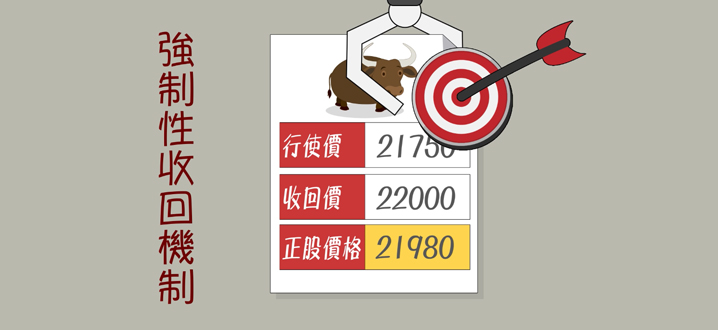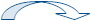•牛熊证攻略 第一节
甚麽是牛熊证？
•牛熊证攻略 第二节
选择牛熊证时应注意
•牛熊证攻略 第三节
牛熊证收回机制及结算方法
II. 牛熊证收回机制

#### 上午被收回#### 下午被收回#### R类牛证例子

没触及收回价 触及收回价

(I)没触及收回价

=(正股结算价 － 牛证行使价) / 换股比率
=(\$62 - \$50) / 10
=\$1.2

(II)触及收回价

=(正股最低价 － 牛证行使价) / 换股比率
=(\$56 - \$50) / 10
=\$0.6

#### R类熊证例子

没触及收回价 触及收回价

(III) 没触及收回价

=(熊证行使价 - 正股结算价) / 换股比率
=(\$68 - \$62) / 10
=\$0.6

(IV) 触及收回价

=(熊证行使价 - 正股最高价) / 换股比率
=(\$68 - \$66) / 10
=\$0.2